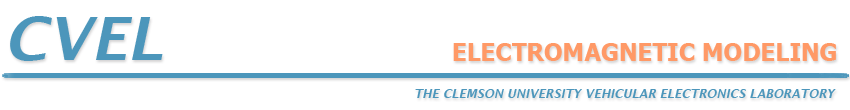# The Partial Element Equivalent Circuit Method

The Partial Element Equivalent Circuit (PEEC) Method was developed by Ruehli [1, 2] in the 1970s and 1980s. This electromagnetic modeling technique does not solve Maxwell's equations directly. Instead, it models electric field interactions as capacitances and magnetic field interactions as inductances. Metallic geometries are segmented on their surfaces, much as they would be using other integral equation techniques. Dielectrics (other than free space) are segmented throughout their volume. Each segment is then represented as a node in a large circuit model. The currents flowing in these segments are determined by analyzing the circuit using a SPICE-like circuit solver.

This technique has the advantage that it is reasonably intuitive to many electrical engineers and it is easy to integrate the field solver with real circuit elements. Problems can be solved in the time domain or in the frequency domain.

Static modeling with PEEC codes is particularly powerful and intuitive [3-6]. Full-wave modeling requires a circuit solver that can handle time-retarded values of mutual capacitance and inductance. Since this is not a normal feature of SPICE solvers, the circuit solver for full-wave PEEC codes must generally be distributed with the PEEC software.

## References

 A. Ruehli, "Equivalent circuit models for three dimensional multiconductor systems," IEEE Trans. on Microwave Theory and Tech., vol. 22, no. 3, pp. 216-221, Mar. 1974.

 A. Ruehli and H. Heeb, "Circuit models for three-dimensional geometries including dielectrics," IEEE Trans. on Microwave Theory and Tech., vol. 40, no. 7, pp. 1507-1516, July 1992.

 C. Wollenberg and A. Gurisch, "Analysis of 3-D interconnect structures with PEEC using SPICE," IEEE Trans. on Electromag. Compat., vol. 41, no. 4, pp. 412-417, Nov. 1999.

 Y. Cao, Z.-F. Li, J.-F Mao, J.-F Mao, "A PEEC with a new capacitance model for circuit simulation of interconnects and packaging structures," IEEE Trans. on Microwave Theory and Tech., vol. 48, no. 9, pp. 1435-1442, Sep. 2000.

 D. Gope, A. Ruehli, V. Jandhyala, "Solving low-frequency EM-CKT problems using the PEEC Method," IEEE Trans. on Adv. Packaging, vol. 30, no. 2, pp. 313-320, May 2007.

 G. Antonini, "PEEC capacitance extraction of 3-D interconnects," Science, Measurement & Technology, IET, vol. 1, no. 4, pp. 201-209, July 2007.